## Sunday, August 21, 2011

### Moving Average in DAX / BISM Tabular

SQL Server Denali | PowerPivot

Alberto Ferrari already wrote about calculating moving averages in DAX by using a calculated column. I’d like to present a different approach here by using a calculated measure. For the moving average I’m calculating a daily moving average (over the last 30 days) here.

For my example, I’m using the PowerPivot workbook which can be downloaded as part of the SSAS Tabular Model Projects from the Denali CTP 3 samples.

In this post, I’m developing the formula step by step. However, if you are in a hurry, you might directly want to jump to the final results below.

With calendar year 2003 on the filter, date on columns and sales amount (from table Internet Sales) in the details, the sample data looks like this: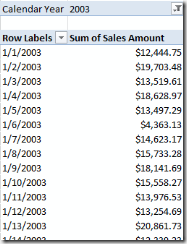In each row’s context, the expression 'Date'[Date] gives the current context, i.e. the date for this row. But from a calculated measure we cannot refer to this expression (as there is no current row for the Date table), instead we have to use an expression like LastDate('Date'[Date]).

So, in order to get the last thirty days we can use this expression

DatesInPeriod('Date'[Date],LastDate('Date'[Date]),-30,DAY)

We can now summarize our internet sales for each of those days by using the summarize function:

Summarize(
DatesInPeriod('Date'[Date],LastDate('Date'[Date]),-30,DAY)
,'Date'[Date]
, "SalesAmountSum"
, Sum('Internet Sales'[Sales Amount])
)

And finally, we’re using the DAX function AverageX to compute the average of those 30 values:

Sales Amount (30d avg):=AverageX(
Summarize(
DatesInPeriod('Date'[Date],LastDate('Date'[Date]),-30,DAY)
,'Date'[Date]
, "SalesAmountSum"
, Sum('Internet Sales'[Sales Amount])
)
,[SalesAmountSum]
)

This is the calculation that we are using in our Internet Sales table as shown in the screenshot below: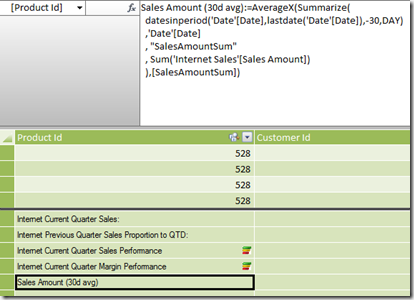When adding this calculation to the pivot table from above, the result looks like this: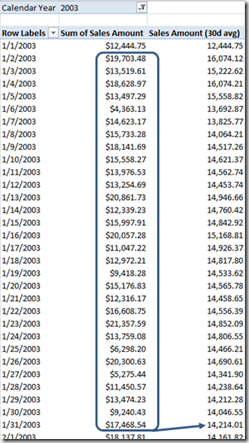Looking at the result it seems that we don’t have any data prior to January 1, 2003: The first value for the moving average is identical to the day value (there are no rows before that date). The second value for the moving average is actually the average of the first two days and so on. This is not quite correct but I’m getting back to this problem in a second. The screenshot shows the computation for the moving average of at January 31 as the average of the daily values from January 2 to 31.

Our calculated measure also works fine when filters are applied. In the following screenshot I used two product categories for the data series: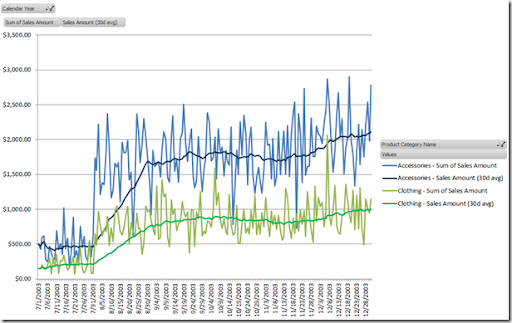How does our calculated measure work on higher aggregation levels? In order to find out, I’m using the Calendar hierarchy on the rows (instead of the date). For simplicity I removed the semester and quarter levels using Excel’s pivot table options (Show/Hide fields option).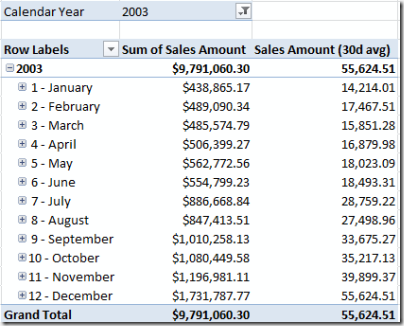As you can see, the calculation still works fine. Here, the monthly aggregate is the moving average for the last day of the specific month. You can see this clearly for January (value of 14,215.01 also appears in the screenshot above as the value for January 31). If this was the business requirement (which sounds reasonable for a daily average), then the aggregation works fine on a monthly level (otherwise we will have to fine tune our calculation and this will be a topic of am upcoming post).

But although the aggregation makes sense on a monthly level, if we expand this view to the day level you’ll see that our calculated measure simply returns the sales amount for that day, not the average of the last 30 days anymore: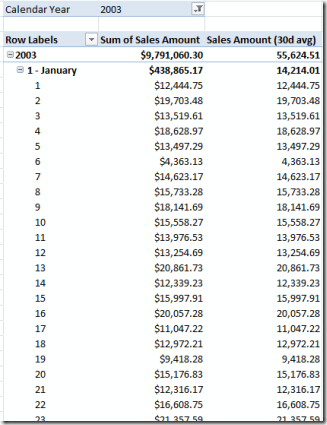How can this be. The problem results from the context in which we calculate our sum, as highlighted in the following code:

Sales Amount (30d avg):=AverageX(
Summarize(
datesinperiod('Date'[Date],LastDate('Date'[Date]),-30,DAY)
,'Date'[Date]
, "SalesAmountSum"
, Sum('Internet Sales'[Sales Amount])
)

,[SalesAmountSum]
)

Since we evaluate this expression over the given dates period, the only context that is overwritten here, is 'Date'[Date]. In our hierarchy we’re using different attributes from our dimension (Calendar Year, Month and Day Of Month). As this context is still present, the calculation is also filtered by those attributes. And this explains why we the current day’s context is still present for each line. To get things clear, as long as we evaluate this expression outside of a date context, everything is fine as the following DAX query shows when being executed by Management Studio on the Internet Sales perspective of our model (using the tabular database with the same data):

evaluate (
Summarize(
datesinperiod('Date'[Date],date(2003,1,1),-5,DAY)
,'Date'[Date]
, "SalesAmountSum"
, Sum('Internet Sales'[Sales Amount])
)
)

Here, I reduced the time period to 5 days and also set a fixed date as LastDate(…) would result in the last date of my date dimension table for which no data is present in the sample data. Here is the result from the query: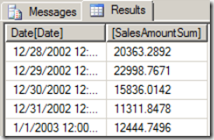However, after setting a filter to 2003, no data rows outside of 2003 will be included in the sum. This explains the remark above: It looked like we only have data starting from January 1, 2003. And now, we know why: The year 2003 was on the filter (as you can see in the very first screen shot of this post) and therefore it was present when calculating the sum. Now, all we have to do is to get rid of those additional filters because we’re already filtering our results by Date. The easiest way to do so, is to use the Calculate function and apply ALL(…) for all attributes for which we want to remove the filter. As we have some of those attributes (Year, Month, Day, Weekday, …) and we want to remove the filter from all of them but the date attribute, the shortcut function ALLEXCEPT is very useful here.

If you do have an MDX background you will wonder why we don’t get a similar problem when using SSAS in OLAP mode (BISM Multidimensional). The reason is that our OLAP database has attribute relationships, so after setting the date (key) attribute, the other attributes are automatically changed too and we don’t have to take care about this (see my post here). But in the tabular model we don’t have attribute relationships (not even a true key attribute) and therefore we need to eliminate unwanted filters from our calculations.

So here we are with the …

Final results

Sales Amount (30d avg):=AverageX(
Summarize(
datesinperiod('Date'[Date],LastDate('Date'[Date]),-30,DAY)
,'Date'[Date]
, "SalesAmountSum"
, calculate(Sum('Internet Sales'[Sales Amount]), ALLEXCEPT('Date','Date'[Date]))
)
,[SalesAmountSum]
)

And this is our final pivot table in Excel: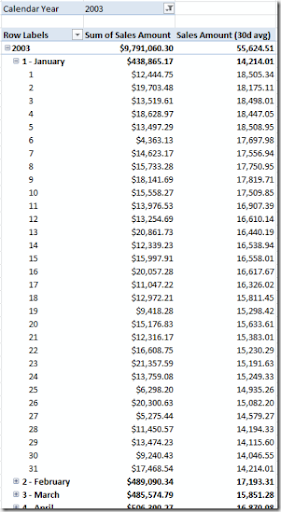To illustrate the moving average, here is the same extract of data in a chart view (Excel):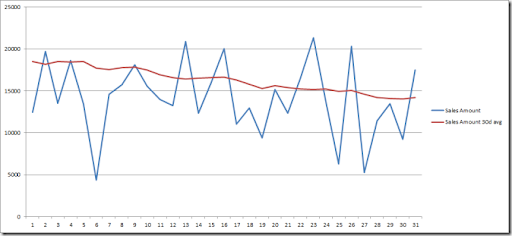Although we filtered our data on 2003 the moving average for the first 29 days of 2003 correctly takes the corresponding days of 2002 into account. You will recognize the values for January 30 and 31 from our first approach as these were the first days for which our first calculation had a sufficient amount of data (full 30 days).

## Saturday, August 13, 2011

### Semi additive measures in DAX / BISM Tabular

SQL Server Denali | PowerPivot

Semi additive measures, i.e. measures that have to be aggregated differently over different dimensions, are commonly used in BI solutions. One example could be stock levels. Of course we don’t want to sum them up over time, but only over product, location etc. For the time, a different aggregation is used, for example average or last value.

The following example shows how to implement some of the most commonly used semi additive measures in DAX.

In my example I’m using PowerPivot (Denali edition), but the same calculations can be used in a BISM Tabular model in Visual Studio.

In order to keep things simple, I’m using just a short table of test data: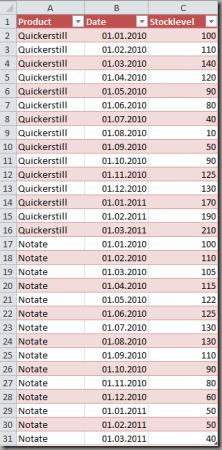As you see, we only have two products with monthly stock levels in 2010 and 2011.

Although not needed for my semi additive measures, I created additional columns in my PowerPivot sheet for convenient reasons: Year, Month, Day (using the corresponding DAX-function with the same name). I also set the newly created columns, as well as the Stocklevel column to hidden (it makes no sense to sum up the stock level). Although the date information is kept in the same table as the data to keep things simple for this example, I encourage to build a separate date dimension table here (similar idea as with a date dimension in a multidimensional model).

Finally, I created a hierarchy named ‘Calendar’ on my newly created date columns: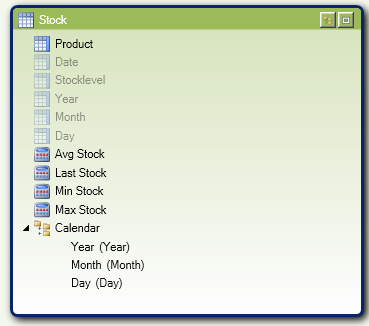Average (over time)

Let’s start with an easy one, the average over time. Since we can easily compute the distinct count of our date values, we can simply add up the stock level and divide it by the distinct count. In my example the formula looks like this:

Avg Stock:=Sum([Stocklevel])/DISTINCTCOUNT([Date])

Last value (over time)

In order to compute the last value, the DAX function LASTDATE comes in handy. Here is the formula:

Last Stock:=CALCULATE(SUM([Stocklevel]),LASTDATE('Stock'[Date]))

Min/Max value (over time)

For min/max we have to be a little bit more tricky. In the approach I’m showing here, I’m grouping the table by date by using the SUMMARIZE function and the SUM aggregation. Then I’m using the function MINX or MAXX to find the minimal or maximal value.

Here are the two formulas:

Max Stock:=MINX(SUMMARIZE('Stock','Stock'[Date],"SumByDate",SUM('Stock'[Stocklevel])),[SumByDate])

Max Stock:=MAXX(SUMMARIZE('Stock','Stock'[Date],"SumByDate",SUM('Stock'[Stocklevel])),[SumByDate])

To understand these formulas you can see the effect pretty well after restoring the PowerPivot workbook to a SSAS server in tabular mode. After doing so, we can create a query to show the result of the inner SUMMARIZE function using this DAX query:

evaluate(
SUMMARIZE('Stock','Stock'[Date],"SumByDate",SUM('Stock'[Stocklevel]))
)

Here’s the result: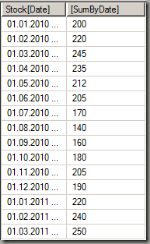The MinX or MaxX function simply takes the lowest/highest value from this table.

Now let’s see, how this looks like in Excel. The following screenshot shows the calculations in my PowerPivot sheet: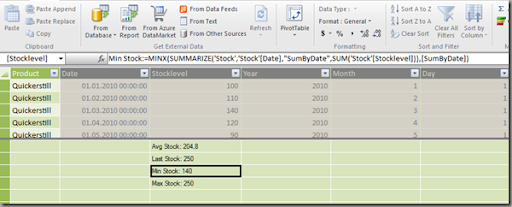Here’s the result in Excel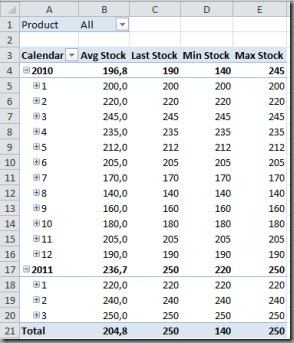And of course, the aggregations also work correctly when filtering the data as shown below (single select on product and multi select on months):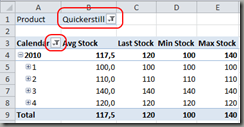Another cool feature is that besides DAX we can still use standard MDX to query our SSAS tabular model, for example:

select
{[Measures].[Avg Stock],[Measures].[Last Stock],
[Measures].[Min Stock],[Measures].[Max Stock]} on 0,
[Stock].[Calendar].[Year] on 1
from [Model]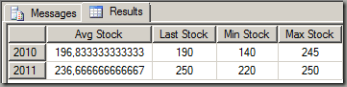A final word about empty (missing) rows: The above calculations need a value of zero as the information that there is no stock at that month. If the value is left blank (no source data row at all), the month itself is treated as missing (interpretation more like we didn’t have this product in our portfolio at all).